# Further Application — The Catenary Problem

So, we derived the Euler-Bernoulli Beam equation, and solved some beam bending problems, but in the process we developed some general tools, so we really should try to use them for more things, if possible.

We’ll start with the catenary problem.  Catenary comes from the latin word for chain.  A chain is hanging from two fixed points.  What shape does it take?

Because this is a chain, you can rotate the links relative to each other freely.  They are not “hinges” with elastic energy.  We also assume the chain does not stretch, so in fact there is no elastic energy whatsoever.  There is only the potential energy due to the chain’s own weight (which we will treat as itself a length, since they must be proportional).  So, the solution is the path that minimizes potential energy.

We’ll say one end of the chain is at (0,0) while the other is at (a,0).  Of course, a must be less than or equal to L (the chain length) for this to make sense.

The function to take the extreme of is:The first term is the potential energy, the second (with the first Lagrange multiplier) references that the end of the chain must go through x=a, and the third (second Lagrange multiplier) references that it also must go through y = 0.

We can find the extreme in several ways.  One is to do it numerically:  Pick some large n (maybe 60), and use Excel to fit all 60 angles.  Since Excel can find extremes itself, we don’t even need to take the gradient of that function.

If we do need to take the gradient, it is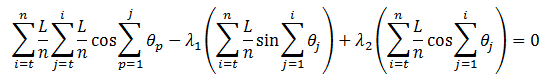[Equation 1]

Another thing we can do is, like last time, do a small angle approximation.  Note that this can only be done after we take the gradient:  Otherwise, the cosine would have differentiated to 0 (rather than sin x ~ x), and we would have no angles to solve for!

Another way of looking at it is that in the original function, sin x ~ x, cos x ~ 1 – x^2/2 (ie., take two terms for the cosine rather than just one).  In fact, we will have to come back to this later.

One thing that is trickier for this problem, compared to beam bending, is that the thetas are not uncoupled.  However, it is fairly simple to invert the system.

Let’s call the middle term (ignoring lambda and L/n) g(t).  Then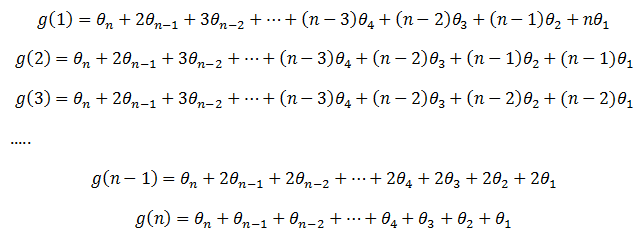These can be inverted withThe other sums are easily evaluated as before, so we get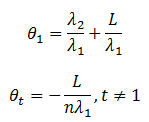Plug into the y=0 condition and we get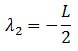Plug that into the x=a condition and we getNow plugging back into x and y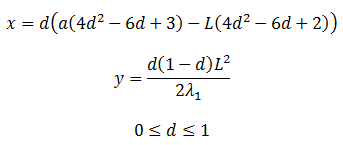One point of discussion:  Note that Lambda-1 involves taking a square root.  You can take it positive or negative, which also changes the sign of y.  This is because this procedure finds the extreme of a function, and the function has two: One minimum in potential energy when it hangs down, and one maximum when it hangs upward but otherwise with the same shape.

Now let’s try to solve this problem exactly.

Go back to Equation 1.  Call it W(t).  For example,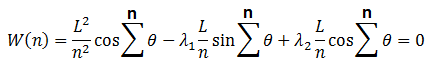In general, we can rewrite it asIn the small angle case, we made progress by subtracting these expressions, so let’s do that again: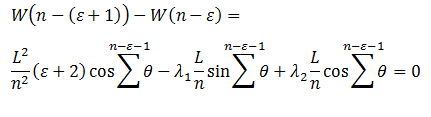With a little re-writing:Okay, let’s think about the physical meaning of this equation.

The first term is a length.  As epsilon increases, it goes from zero to the total length of the chain (with maybe an off-by-one or -two error that becomes insignificant as n tends to infinity).  The length is from the end of the curve (x=a) to whatever point the angle index epsilon refers to.  Let’s say that’s x=c.

The tangent of a sum of angles is the derivative at that point (again, x=c).

So we have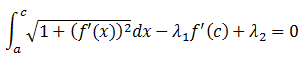(I may be acting cavalier about whether or not there is a minus sign on the first term / whether the integral goes from a to c or c to a, but I don’t think it matters)

Differentiate with respect to c:Set g = f’, treat dg/dx as a fraction, rewrite and integrate:So now we have to do that integral. You might just recognize it.  Wolfram Alpha gives it directly.  The TI89 sort of can do it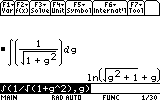but you still need to recognize it as a hyberbolic function.  It does recognize a variant as a trig functionAnyway, so we havek is an integration constant.

Integrate again to get f: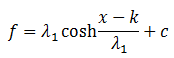c is an integration constant (different from the earlier c).

This mostly solves the problem.  k and c can be chosen to fit the boundary conditions.  If the curve goes through (0,0) and (a,0), they are k = a/2, c = -lambda*cosh(k/lambda).

Lambda1 is chosen to make the entire length of the curve L; however, I think that condition leads to a transcendental equation!

What happened to Lambda2?  I think that just came up because we were measuring the arc length from one end rather than the middle.

The first tab is to solve it numerically.  This is an example of settings for that.  There is also a comparison to the analytic result, and they should always fit well.  You may need to adjust (or solve for) the parameter in L4 to make the analytic length in Q2 equal to 1.

The next tab compares the exact result to the small angle result.  The parameter to change is a, the distance the two ends are held from each other.

This tab supposedly solves for the parameter itself, but if you get a weird value or the analytic cosh is flipped upside down, you may need to enter a different guess into M2.

At a=0.95, the exact and small angle solutions are extremely close:At x=0.9, they are still close, but the difference is becoming visible:At x=0.6, the two curves are quite different:________________________________________

I just noticed the above graphs are labeled redundantly, “hyperbolic cosh”

________________________________________

I think there was one loose end I may have wanted to address.  When we derived the Euler-Bernoulli beam equation, we assumed there were no other boundary conditions.  What happens if we do that here?

I think it eventually leads to dx/dy = 0, meaning the chain is hanging straight down, which I think makes sense.  So you need to (in principle — note that the information that the curve goes through the exact point (a,0) goes away when you take the gradient) have some other condition on the chain to keep it from just hanging down.